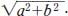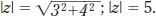NextPrevious

# What is the absolute value of a number?

The absolute value of a real number is the number stripped of any negative value. Therefore, the absolute value of a number will always be greater than or equal to zero. (Formally, the absolute value is considered the distance of a number from zero on a number line.) The symbol for “absolute value” is the number inside two parallel vertical lines (| |). For example, the absolute value of x is given by |x|. If the number is negative within the absolute value sign, it will automatically become positive. In numerical form, |3| equals 3 and |-3| equals 3.

When discussing complex numbers, the absolute value often means squaring the numbers, then taking the square root of those numbers. For example, the common way of writing complex equations is z = a + bi; the absolute value of z becomes. For instance, if z = 3 - 4i, thenClose

This is a web preview of the "The Handy Math Answer Book" app. Many features only work on your mobile device. If you like what you see, we hope you will consider buying. Get the App